### Home > MC2 > Chapter 6 > Lesson 6.2.4 > Problem6-109

6-109.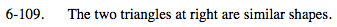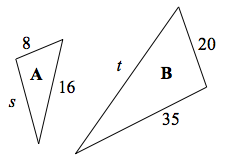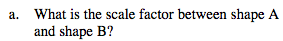Which corresponding sides in A and in B are both known?

What do you need to multiply 8 by to get 20?

$\frac{20}{8}=2.5$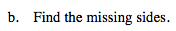Identify which sides in A correspond to which sides in B.

Since 16 corresponds to t and s corresponds to 35, multiply 16 by the scale factor to find t, and divide 35 by the scale factor to find s.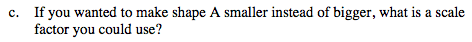The scale factor is what you multiply by to increase or decrease the size of a shape.

If a scale factor greater than one created a larger triangle, then a scale factor less than one must create a smaller triangle.
Note that a scale factor cannot be negative because a negative side length is nonexistent.